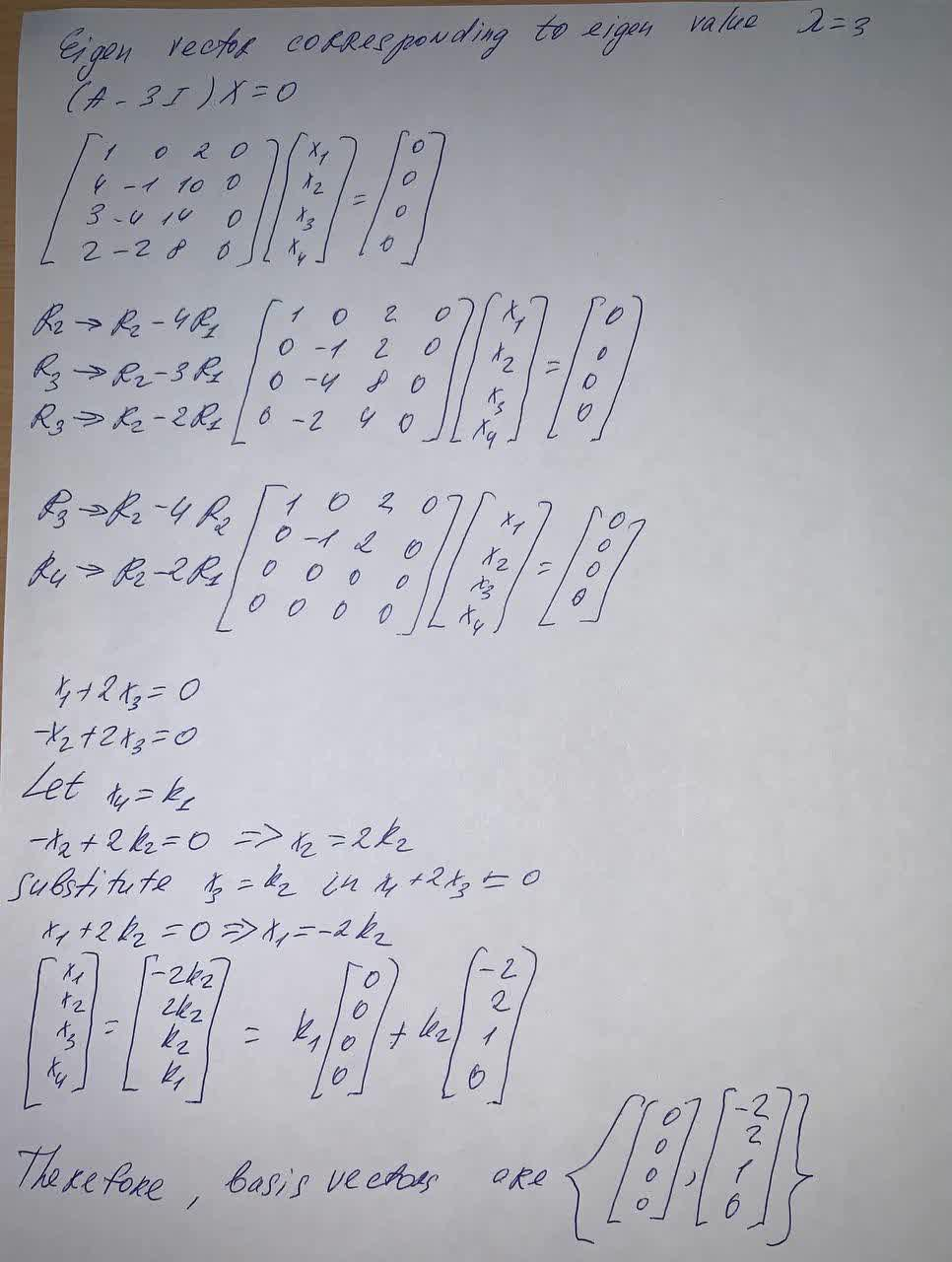Question# Find a basis for the eigenspace corresponding to the eigenvalue of A given below. A=\begin{bmatrix}4 & 0&2&0 \\4 & 2&10&0\\3&-4&17&0\\2&-2&8&3 \end{bmatrix} , \lambda=3

Parametric equations, polar coordinates, and vector-valued functions
ANSWERED$$A=\begin{bmatrix}4 & 0&2&0 \\4 & 2&10&0\\3&-4&17&0\\2&-2&8&3 \end{bmatrix} , \lambda=3$$